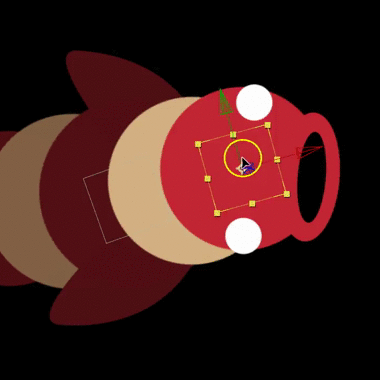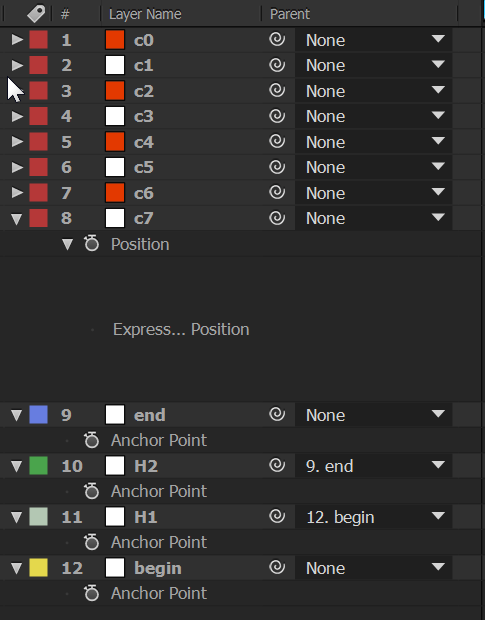# Using Beziér curves to animate layers in After Effects.

It’s great to be able to animate a stack of layers using familiar Beziér handles. This is great for flexible spines, caterpillars, snakes, or in the example I’m working on: swimming fish.

It creates an easy to use rig, that allows you to easily create fluid motion with minimal controls.

I’ve done this a couple of times, and each time I’ve had to consult Wikipedia for the formula and then turn that into useable expressions. So to prevent me having to reinvent it another time, here’s how it’s done…

#### First I made my controls

There are four controls: the beginning and end points, and two handles to define the curve. They’re all nulls, but you could use anything. In the example above the beginning is yellow, the handles are ‘sea foam’ and green and the end is blue.

To make editing easier I parented the handles to the end points—if you’re used to Beziér controls in most design apps this is the way they normally work, with the point having handles that are attached to it. It also allows me to rotate the end points to twist the spline, meaning I can mostly just move and rotate my end points to control the whole thing.

#### Next I made my layer stack

These are the layers that are going to be animated. In this case it is a bunch of solids with circular masks. I named them—and this is important—as c1, c2 c3 etc. They’re above the controls in the timeline—this too is important for the way I’ve implemented the expression. They don’t have to be the same, in this example they all have different scale, and they’re based on two different coloured solids.#### Time to get mathsy

Don’t worry, you can skip this bit if you’re allergic to maths.
A Beziér curve is defined by this equation:

B(t) = (1 – t)3P0 + 3(1 – t)2tP1 + 3(1 – t)t2P2 + t3P3 ,
0 ≤ t ≤ 1

Where:

• t is the distance along the curve, expressed as a value from 0 to 1;
• P0 is the start,
• P1 and P2 are the handles and
• P3 is the end point.

P0 P1, P2 and P3 are all vectors, aka two- or three-dimensional arrays or matrices. Now to turn that into a useable expression.

#### First we define the points

P0 and P3 e easy; they’re the position of these points

```p0=thisComp.layer("begin").transform.position;
p3=thisComp.layer("end").transform.position;
```
##### Next, the handles.

Because I parented the handles to the end points we need to get their world position, which we do with a layer space transform, in this case toWorld:

```p1=thisComp.layer("H1").toWorld(thisComp.layer("H1").transform.anchorPoint); p2=thisComp.layer("H2").toWorld(thisComp.layer("H2").transform.anchorPoint);
```

A layer’s `toWorld()` method returns the position of a given point on that layer with respect to the the world. Here we’re getting each handle’s anchor point in world terms.

##### Next we definet

This is a value from 0 to 1, There are lots of other ways you could do this, in this case I use the layer’s name to drive it. You could also use the layer’s index, but the method I used has the advantage that you don’t need to keep the layers in order, and you can have more than one layer at the same point. I take advantage of javascript’s sloppiness with typing. Strings can become numbers if you treat them as numbers, without any to-do. So I use the numerical suffix of the layer’s name to define its position along the curve (I’ve updated it to work with any number of layers).

`<layer>.name` returns the name of the layer, which is a string. We use the JS string method `match()`to find a numerical suffix in the name, using regular expressions. We’re looking for one or more integers, which in regex is `[0-9]+` at the end of the line, which in regex is `\$`. So the regex we use is `/[0-9]+\$/` This will return an string containing any contiguous numbers at the end of the layer name integer, eg “layer4-copy 123” returns “123”. This is still a string, but like I said Javascript is just hot-glue and duct tape, so if we treat it like a number it will act like a nimber. We could use `parseInt()` to be sure, but who has the time?

Then we divide the integer by the number of layers in the stack, which we find by getting the index of the end point (that’s why the layer stack has to be above the control points). This makes sure that 0<t<1 or in other words it normalises it to a value between 0 and 1.

```t=(thisLayer.name.match(/[0-9]+\$/) / (thisComp.layer("end").index-2);
```

### Now for the real hoo-hah.

This is the javascript implementation of the Beziér formula. It’s a bit harder to read, because of all the `Math.pow()` palaver, but what can you do?

```Math.pow((1 - t), 3)* p0 + 3 * Math.pow(1 - t, 2) * t * p1 + 3 * (1 - t) * Math.pow(t, 2) * p2 + Math.pow(t, 3) * p3;
```

You’ll notice that we don’t have to split up the x and y components of any of the points. Remember how p0, p1 etc. were vectors in the Beziér equation? Well you can do vector maths in expressions, as long as you only multiply vectors by scalars (normal, single component numbers like integers and decimals), and you only add vectors to other vectors. One of the advantages of this is that the expression will work just as well on a 2D or 3D layer. Magic.

### TL;DR

if your eyes are glazing over, here’s where you get to copy and paste.

TODO: a script to apply this all automagically. For now, set up your layers as described, and apply this to the animated layer’s position property:

```p0=thisComp.layer("begin").transform.position; p1=thisComp.layer("H1").toComp(thisComp.layer("H1").transform.anchorPoint); p2=thisComp.layer("H2").toComp(thisComp.layer("H2").transform.anchorPoint);
p3=thisComp.layer("end").transform.position;
t=(thisLayer.name.match(/[0-9]+\$/)) / (thisComp.layer("end").index-2);
Math.pow((1 - t), 3)* p0 + 3 * Math.pow(1 - t, 2) * t * p1 + 3 * (1 - t) * Math.pow(t, 2) * p2 + Math.pow(t, 3) * p3;
```

### Here’s the script in action:

1.This is great, lots of fun! I’m trying to add more than 10 layers and when I change your code to use “split(0-n)” as you suggest, AE gives me the error “n is not defined.” I’m not a good with coding but would very much like to try this with more than 10 layers 🙂

1.Hi Mark, thanks for your post. I’ve updated it to take advantage of the modern JS engine. The code should now work with any number of layers without any modification.

2.